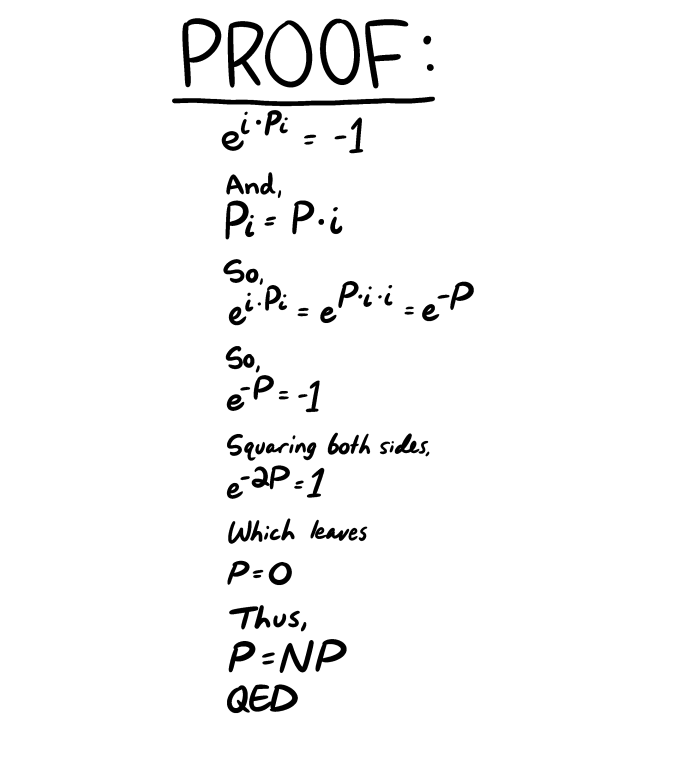## Funny

Today's SMBC:Somewhere Leonhard Euler and Kurt Gödel are having a good laugh.

1. ### Sili said,

November 8, 2015 @ 9:34 am

I suppose if I insisted it should at least be \pi, then that would just get incorporated into some kind of ungodly ratio.

[(myl) That's "matrix left divide" in Matlab…]

2. ### D.O. said,

November 8, 2015 @ 9:55 am

Well, getting from exp(-2P)=1 to P=0 requires some nerve…

3. ### Jonathan said,

November 8, 2015 @ 10:25 am

If this is gonna be that kind of party, we can divide P = NP on both sides by P, which yields N =1, so we reject the analysis for insufficient data.

4. ### chris said,

November 8, 2015 @ 10:37 am

@Jonathan: even though it was already stated that P=0?

@D.O. Actually, of all the things that are wrong with this "proof", I don't think that's one of them. IIRC, exponentiation is one-to-one, so if exp(-2P)=1=exp(0), then -2P=0.

…hmm, now that I think about it, maybe it's only one-to-one over the reals. So the issue may be more complex than I thought.

5. ### James said,

November 8, 2015 @ 5:32 pm

Chris:
You mean, more than you imagined.

6. ### Guy said,

November 9, 2015 @ 3:49 pm

If we interpret P as representing a complex number, then all the steps are indeed valid up until P=0, which does not follow. It would follow if we knew that P was a real number, but no real number would satisfy the first equation. More generally, the first, third, and fourth equations are true for complex P if and only if P is an odd integer times pi (the constant) times the imaginary unit. The fifth is true for any integer times pi times the imaginary unit (we lose information by squaring both sides, since squaring is not injective).

7. ### Jongseong Park said,

November 9, 2015 @ 3:52 pm

Right, the solution to exp(x) = 1 for x is the set of integer multiples of 2πi. This means that if exp(-2P) = 1, then P could be any integer multiple of πi.

8. ### Mark S said,

November 14, 2015 @ 8:58 am

Great to see how many mathematicians follow Language Log!

9. ### BZ said,

November 16, 2015 @ 5:20 pm

It also doesn't work if you plug P=0 back into the equation, because it would imply e^0=-1